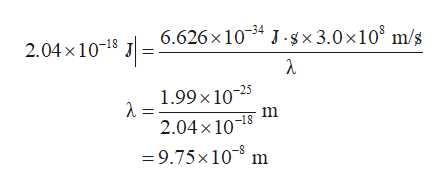# What is the wavelength λ of the photon that has been released in Part B?ΔE = −2.04×10−18 JThe energy of the photon emitted by the electron is related to its wavelength by E=hcλwhere λ is the wavelength in meters, 6.626×10−34 J⋅s is Planck's constant, and 3.00×108 m/s is the speed of light in a vacuum.

Question
4877 views

What is the wavelength λ of the photon that has been released in Part B?

 ΔE = −2.04×10−18 J

The energy of the photon emitted by the electron is related to its wavelength by

E=hcλ

where λ is the wavelength in meters, 6.626×10−34 J⋅s is Planck's constant, and 3.00×108 m/s is the speed of light in a vacuum.

check_circle

star
star
star
star
star
1 Rating
Step 1

The relation between energy and wavelength of a radiation is given by Planck-Einstein’s relation, stated below as expression (1); where, E is the energy, h is the Planck’s constant, c is the speed of light, and λ is the wavelength.

Step 2

The negative sign of energy in -2.04 × 10-18 J signifies that the energy is released in the process. In order to calculate the wavelength of the photon, the mode...help_outlineImage Transcriptionclose6.626 x 1034 Jgx3.0 x 108 m/s 2.04x10-18 1.99 x 1025 λ- 2.04x 1018 =9.75x 10 m fullscreen

### Want to see the full answer?

See Solution

#### Want to see this answer and more?

Solutions are written by subject experts who are available 24/7. Questions are typically answered within 1 hour.*

See Solution
*Response times may vary by subject and question.
Tagged in